# LET Review 2023: Math: How to Solve Work Problems

Work problems often show up in the teacher’s board for the Mathematics Specialization test and in the General Education Tests. This type of problem may look like this:

Person A can paint a room alone in 5 hours. Person B takes 8 hours to paint the same room. How long will it take Persons A and B to paint the same room together?

When you see a problem similar to this, you only need to remember one formula:

### Work Problems Formula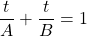In the formula,refers to the time they will spend painting the room together. The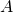refers to the time that person A spends to paint the room alone. In this case,is equal to 5. The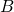refers to the time that person B spends to paint the same room alone. In this case,is equal to 8.

### Given:= time they will spend painting the room together (Unknown)= time that person A spends to paint the room alone= time that person B spends to paint the same room alone

If we substitute the variables with their respective values, we should get this equation.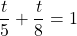After substituting the variables with their respective numerical values, we can now start solving for.
To solve this problem, we will need to apply the rules for adding fractions. Just click the link if you need to review the process: Adding Fractions Review
To solve for, we need to addto. In this case, the lowest common denominator is 40. To add the fractions, we will need to use 40 as the denominator of the new fraction. Our process should now look like this: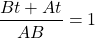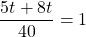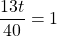At this point, we will need to multiply both side of the equation by 40 to remove the fraction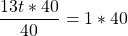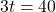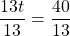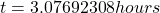Now that you have the answer, you will need to choose the answer in your LET questionnaire that is closest to the answer.

### 2nd Type of the Work Problem

There are many variations of the Work Together Problem. Sometimes,is given and you need to look for eitheror. Here is an example of this variation:

Jamie and Bea can make one hundred hotdog sandwiches in four hours. If Jamie can make the same amount of hotdog sandwiches in eight hours, how long will it take Bea to do all the work by herself?

Using the same equation, first you will need to substitute all the given variables with their respective values. In this problem, the substitution goes like this:

### Given:= 4 hours= 8 hours (Jamie’s working time)= Unknown (We are looking Bea’s time)

The equation goes like this: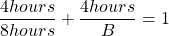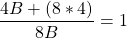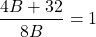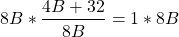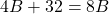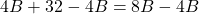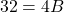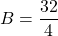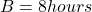The examples in this article are only two of the simple variations of the work problems. After reviewing these problems, you can practice using our online mock tests here: Click Here to Practiceon Work Problems.
We hope these examples will help you pass in the upcoming LET. Have fun practicing!

[qsm quiz=4]

### 3 thoughts on “LET Review 2023: Math: How to Solve Work Problems”

1.Ask ko Lang po sa problem #2? San po galing ung -4B?????

•2.